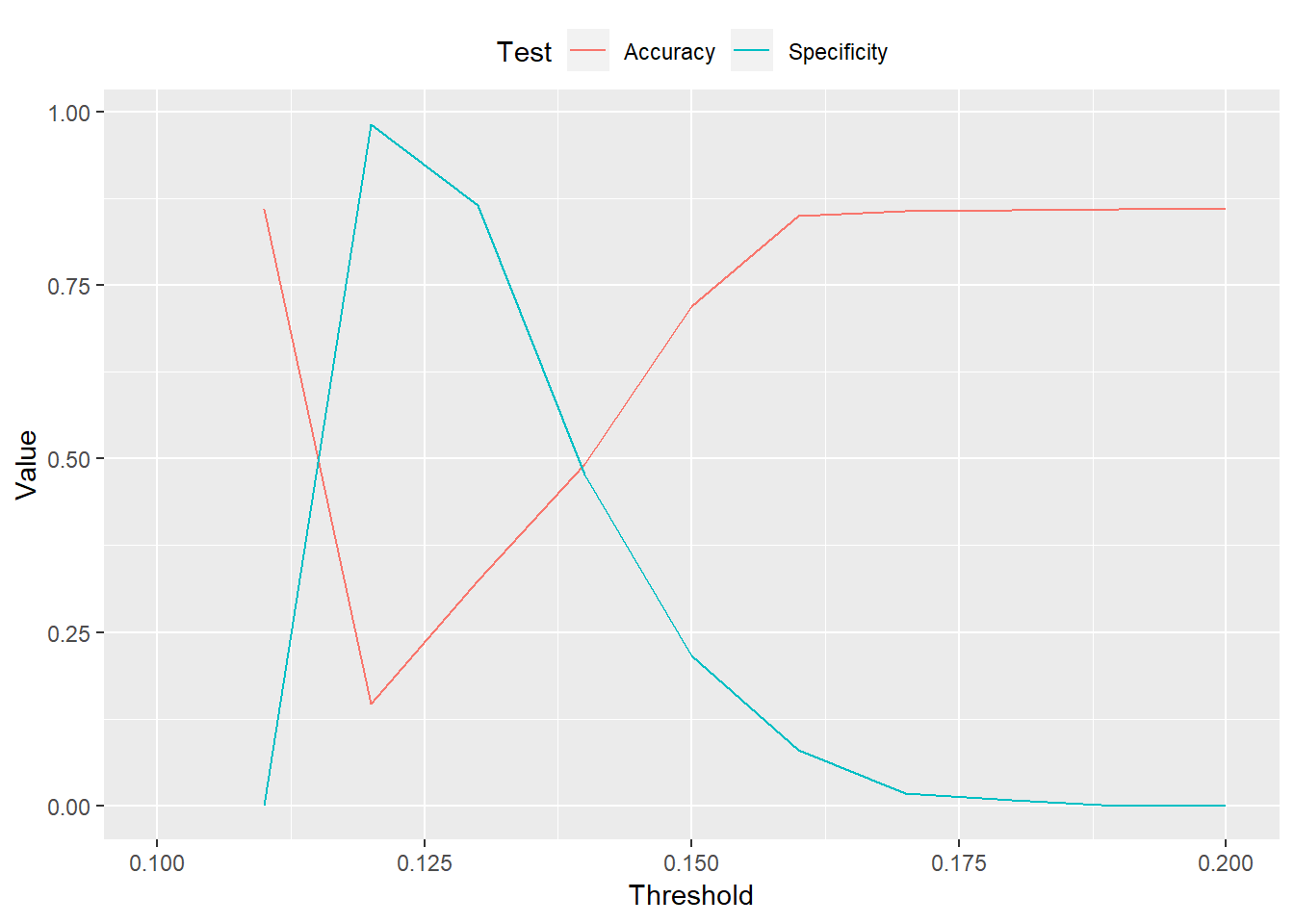Want to share your content on R-bloggers? click here if you have a blog, or here if you don't.

In the last post, we discussed the yield curve, why investors focus on it, and looked at one measure of the curve – the spread between 10-year and 3-month Treasury yields. In this post, we build a model that tries to quantify the probability that the economy is in recession based on the 10-year/3-month spread.

## All models are wrong

A first question to ask is what kind of model should we build? Without getting into too much detail we can build a simple intuition that we need to find a model that can predict whether the economy is in a recession or not. That is kind of like a yes or no state. Without even worrying about the math a model that assumes some sort of linear relationship will probably be inaccurate; after all, lines are supposed to extend indefinitely and recesssions don’t (hopefully!). A classification model might be more appropriate. But which one? Either you’re in a recession or not. Of course, it is possible that parts of the economy exhibit recession-like characteristics. But we’re using the standard defintion of two quarters of negative GDP growth. Additionally, it wouldn’t be intuitively straightforward to predict a recession based on how close the yield curve is to a negative number – a nearest neighbor type model.

Hence the model that makes the most sense to describe the data is a logistic model. We won’t go into the math here. Suffice it to say that a logistic regression model which yields values between 0 and 1 and is reasonably successful in producing probabilities of the form, what is the likelihood of y given x.

### Build the model

For this simple example we use the entire data set beginning in 1953 till the present. Why 1953? That’s when we have the earliest (readily available) data on yields of the maturities we’re looking for. Here’s the graph again for a refresher.As you can see the spread between the 10-year and 3-month yields does tend to dip prior to a recession, as shown by the grey-shaded area. Note that also tends to rebound once the recession is full force. That is probably enough of a reason to doubt that an inverted (negative) spreaad will be a great predictor of a recession without some manipulation of the data. Recall from the first post that the spread was negative only about 12% of the time economy was in recession.

In this first pass, we don’t change the spread data; we simply compare the level of the spread to whether the economy is in recession (coded as a 1 in the data) or not (a 0). This outputs a probability estimate for whether the economy is in recession or not, which we graph against the actual recession.Not that great an outcome. But the visual can’t always give us the best picture of goodness-of-fit. We need a way to score how well the yield curve explains recessions. To do that we look at table of the output as we did in the first post.

In this instance, instead of comparing the presence of an inverted yield curve against the presence of a recession, we compare the probability of a recession against an actual recession. But there’s a problem: the recession is coded as 1 or 0, whereas the probability of recesssion goes from 1 to 0. Tough to count! It’s all well and good to say there’s a 20% chance the economy is in recession. But how do we compare that probability against actual observations? We decide on a cut-off above which we say the model predicts a recession; below, not so much.

This is, of course, entirely arbitrary. And it should also be noted that the model doesn’t actually predict (definitively) whether there’s a recession or not. How we translate the model’s output is as important as the model itself. Naively, we’ll say if the probability is greater than 50%, the model is “suggests” the economy is in recession.

Predicted/Actual No recession Recession
No recession 681 111

Uh oh. If we use 50% as the cut-off, the model predicts no recessions! We spent all this computing power and hours of learning how to build logistic regression models only to yield a result no better than a naive forecast. What a waste.

Not really. What’s the issue? Recessions are uncommon. Recall from the previous post that the economy was in a recession only about 14% (111 months out of 792) of the time since 1953. We’re using a lot of data to forecast a rare event. There are bunch of solutions to this problem. We’ll keep it simple for now.1

Lowering the threshold is the simple solution. Here we say if the model’s probability of recession is above the historical frequency (14%), then we say that the economy is in recession.

No recession Recession
No recession 337 58
Recession 344 53

Let’s look at some metrics:

• Accuracy: 49%
• Specificity: 48%
• False positive rate: 52%

Lowering the threshold produces better results than the previous attempt, but it’s still not much better than a coin flip. If we lower the threshold below 14% then we’re more likely to predict recessions than actually occur. The specificity goes up, but accuracy goes down.

An easy way to see this trade-off is run the range of thresholds through the prediction algorithm and graph the results. As the threshold increases toward the same frequency of recessions, specificity increases, but accuracy decreases.1. We hope to experiment with more advanced solutions in other posts.